# Calculation of the elastic potential energy of a spring when compressed

in StemSocial5 months ago (edited)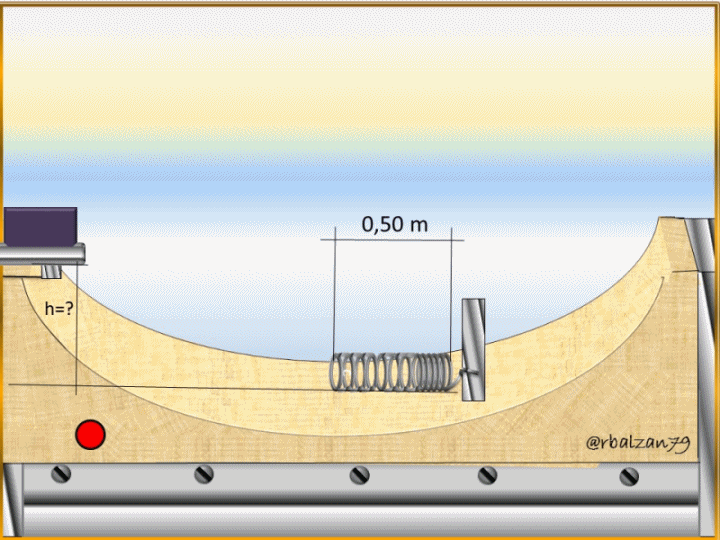Greetings again my dear friends I hope you are all very well, around us there are countless phenomena of vital importance to our existence, one of them, the essential phenomenon of energy, therefore, this time we will relate to the elastic potential energy and, the same, we can say that it is represented by the energy that contains or has a certain system which is linked or associated with an elastic object, such as a spring, which will be our elastic body to analyze.

The elastic potential energy originates due to the application of a certain force to an elastic body and, in this way, it becomes a type of accumulated energy until the force applied to the elastic body or object loses its effect or is no longer applied, thus, the elastic body or object will return to its initial or original shape, all this will generate an effort which is called work.

In relation to the above, I will continue to implement some mathematical formulations in order to know some applications of these formulas through exercises related to everyday activities and that are also very important to provide our students, therefore, my dear friends let's get to know the following exercise.

Exercise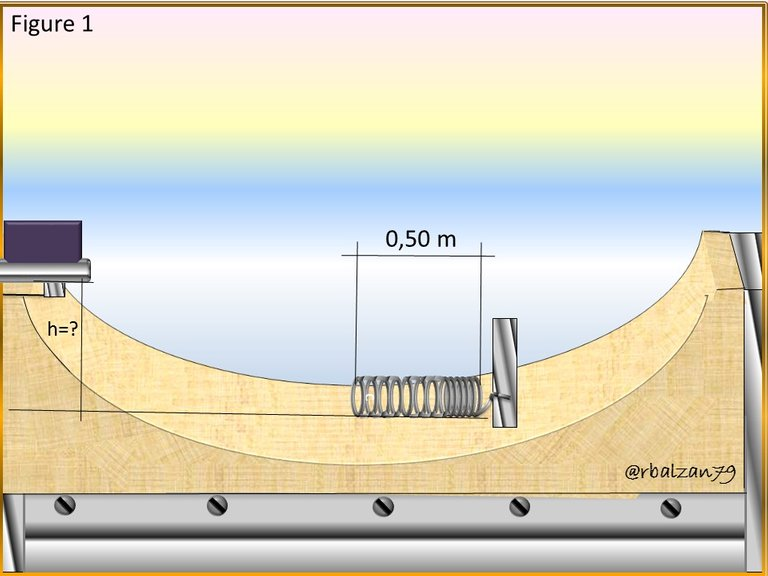If we make a system composed of a spring which is attached at one end to the structure of a ramp and at the other end of the ramp at the top is a mobile, whose mass is 58 kg, the purpose is to release or drop the mobile which is at rest at a certain height as shown in Figure 1 above, and that the spring can brake it, knowing that the elastic constant of the spring is k = 1250 N/m, upon impacting the mobile with the spring, it contracts 15 cm, in relation to the above mentioned, it is important to answer the following questions:

a.- What will be the elastic potential energy of the spring when the 15 cm is compressed?

b.- What was the height of the mobile at the time you let it slide to impact the spring?

Data:
m = 58 kg (Mobile mass).
x = 15 cm = 0,15 m. (Length that the spring has been compressed).
k = 1250 N/m. (Spring elastic constant).
Vo = 0 m/s. (Initial velocity of the mobile).
g = 9,8 m /s2 (Gravity).

Solution

a.- To begin to answer our first question, it is important to know the following formula: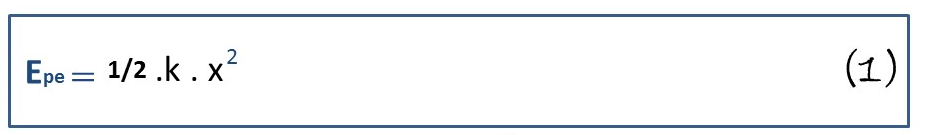It is important to remember the law of conservation of energy, and thus, consider that the total energy of our system will remain constant, our mobile is at total rest, therefore, it has no kinetic energy, so it only has gravitational potential energy because it is at a height (h).

At the moment of letting the mobile slide without friction, this mobile is contained or damped by the spring and in this way, the spring contracts 0.15 m, in this case the energy is, elastic potential, whose value, we can obtain it in the following way implementing formula 1.b.- Now we can move on to the other question, which is related to the height at which we let the mobile slide, therefore, as we expressed that energy is conserved, then, we can say, that all that initial gravitational potential energy was converted or transformed into elastic potential energy, so that: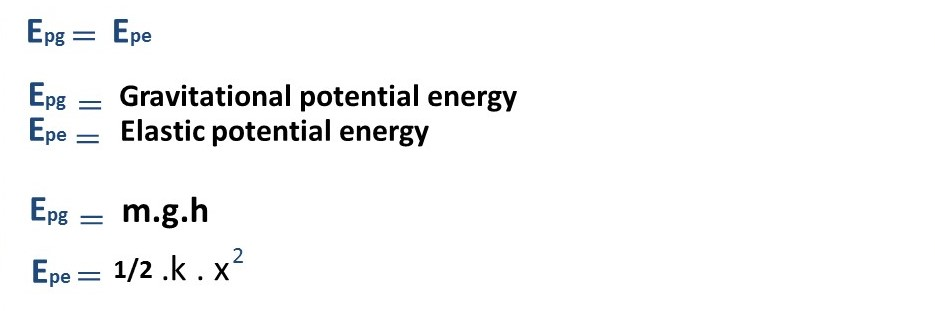Therefore, when substituting we have the following:In this way we can clear our desired unknown, i.e., the height (h) at which the mobile was released to compress the spring 0.15 m.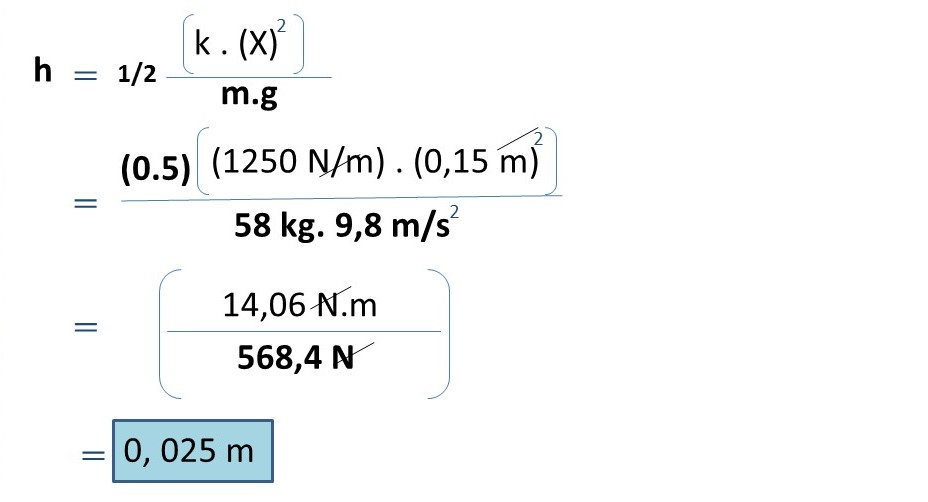This is the value of the height at which the mobile was released indicated in the previous approach, due to this height (0.025 m), the mobile was able to transform the gravitational potential energy into compressed elastic potential energy in the spring.

# Conclusion

In this way we were able to answer the two questions posed, in relation to the first question we were able to calculate the elastic potential energy, which is due to compress the spring, therefore, the accumulated elastic potential energy in this understanding is 14 Joules (J), and this was achieved due to the gravitational potential energy of the mobile since this was released at a height of 0.025m, noting that this accumulated elastic potential energy failed to drive the mobile in reverse, then, the accumulated elastic energy is less than the potential energy of the mobile.

We can always implement any kind of mathematical formulations to understand the behavior of any system next to us, as the one shown in the previous exercise.

Until another opportunity my dear friends.

Note: The images were created by the author using Power Point, the animated gif was created using the PhotoScape application.

# Recommended Bibliographic Reference